JavaFX version: 06/01/2018

# Chapter 121 Programming Exercises

## Exercise 1 — TriangleWrite an program that displays a triangle where the two lower vertices are the centers of the lower left and lower right quadrants and the peak of the triangle is one quarter of the way down the (imaginary) vertical line that divides the scene.

Calculate each of the vertices based on the variables that contain the width and height of the scene.

## Exercise 2 — Four Circles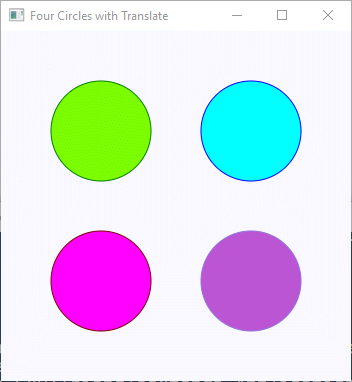Write an program that displays four circles in a square window. Each circle is centered in one of the four quadrants and each circle is a different color of your choice. Pick a radius that is the same for all circles. Calculate the size of the `Scene` based on the radius. Use `Color` constants or define your own colors.

This is the same program as one in the previous chapter. But this time, use the transformations `setTranslateX()` and `setTranslateY()`.

## Exercise 3 — Evergreen Tree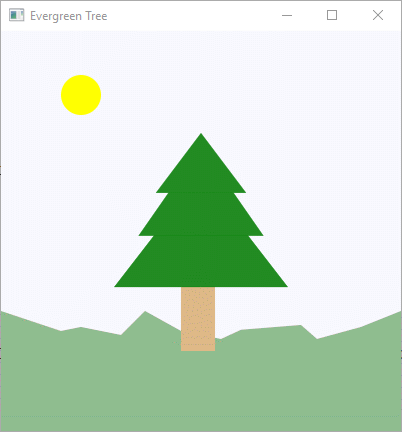Draw an evergreen tree using three overlapping triangles and a rectangle. Remember that the order that the shapes are drawn determines which is geometrically on top of which.

The ground is a `Polygon`. Add yellow sunbeams around the sun, if you want.

## Exercise 4 — Cylinder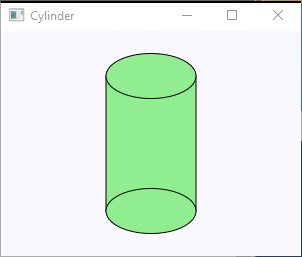Write an program that displays a cylinder viewed from the side. The sides of the cylinder (in the picture) is a rectangle. The top and bottom are ellipses. If you draw them in the right order, the result is a cylinder.

Make the cylinder a `Group` with its top left corner at (0,0). Make the top of the cylinder a `Ellipse` with its center at the center of the top line of the rectangle. Make the radius of the cylinder ends 1.0 and the height of the cylinder 1.0. Then use X and Y translation to move it to the center of the window.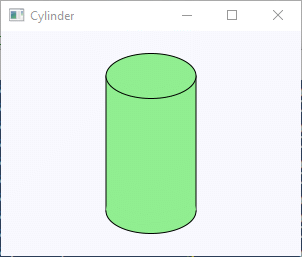You may wish to make only the front edge of the bottom visible. Do this by drawing the bottom `Ellipse` with a transparent stroke and using an `Arc` with `ArcType.OPEN` to draw the bottom edge.

## Exercise 5 — Nested Squares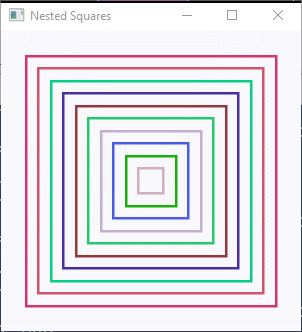Draw `N` nested open squares. The margin around each square is `gap` pixels. The inner-most square is `2*gap` by `2*gap`. Make it easy to change `N` and `gap`. The value for `N` determines the size of the window.

Use `Color.TRANSPARENT` for the fill of each square. Pick a random color for the stroke of each square. Experiment with various ways to change the size of each sequential square.

Put the squares into a `StackPane` so that they are automatically centered.

## Exercise 6 — Random Circles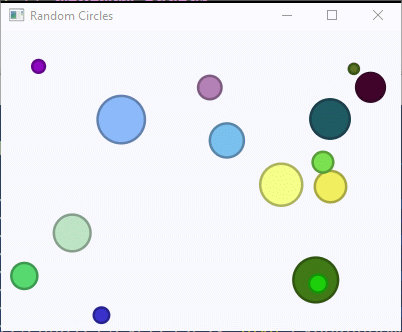Draw N randomly positioned circles each with a random fill color. Make the stroke color a darker version of the fill color. The radius should systematically decrease from a maximum value to a minimum value. Allow the circles to overlap, but draw complete circles, not cut off by the edges of the window. Do this by ensuring that the x and y of each circle is more than the length of the radius away from an edge.

## Exercise 7 — Rotated Ellipses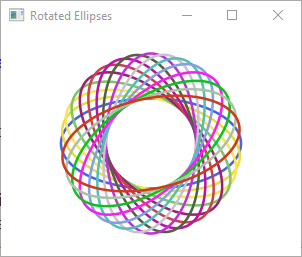Draw N ellipses centered in the window, each rotated by 180/N degrees. Make the fill color TRANSPARENT. Pick a random stroke color for each ellipse.